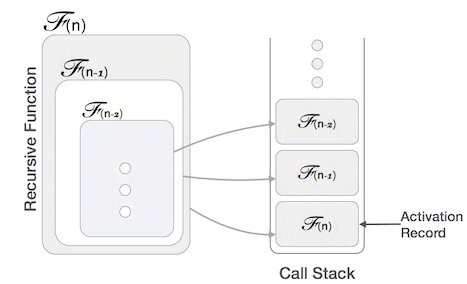# Recursion - Notes | Study GATE Computer Science Engineering(CSE) 2023 Mock Test Series - Computer Science Engineering (CSE)

 Table of contentsIntroductionPropertiesImplementationTime Complexity1 Crore+ students have signed up on EduRev. Have you?

Introduction
Some computer programming languages allow a module or function to call itself. This technique is known as recursion. In recursion, a function α either calls itself directly or calls a function β that in turn calls the original function α. The function α is called recursive function.

Example a function calling itself.

`int function(int value) {   if(value < 1)      return;   function(value - 1);	   printf("%d ",value);   }`

Example − a function that calls another function which in turn calls it again.

`int function(int value) {   if(value < 1)      return;   function(value - 1);	   printf("%d ",value);   }`
Properties

A recursive function can go infinite like a loop. To avoid infinite running of recursive function, there are two properties that a recursive function must have −

• Base criteria − There must be at least one base criteria or condition, such that, when this condition is met the function stops calling itself recursively.

• Progressive approach − The recursive calls should progress in such a way that each time a recursive call is made it comes closer to the base criteria.

Implementation

Many programming languages implement recursion by means of stacks. Generally, whenever a function (caller) calls another function (callee) or itself as callee, the caller function transfers execution control to the callee. This transfer process may also involve some data to be passed from the caller to the callee.

This implies, the caller function has to suspend its execution temporarily and resume later when the execution control returns from the callee function. Here, the caller function needs to start exactly from the point of execution where it puts itself on hold. It also needs the exact same data values it was working on. For this purpose, an activation record (or stack frame) is created for the caller function.This activation record keeps the information about local variables, formal parameters, return address and all information passed to the caller function.

## Analysis of Recursion

One may argue why to use recursion, as the same task can be done with iteration. The first reason is, recursion makes a program more readable and because of latest enhanced CPU systems, recursion is more efficient than iterations.

Time Complexity

In case of iterations, we take number of iterations to count the time complexity. Likewise, in case of recursion, assuming everything is constant, we try to figure out the number of times a recursive call is being made. A call made to a function is Ο(1), hence the (n) number of times a recursive call is made makes the recursive function Ο(n).

## Space Complexity

Space complexity is counted as what amount of extra space is required for a module to execute. In case of iterations, the compiler hardly requires any extra space. The compiler keeps updating the values of variables used in the iterations. But in case of recursion, the system needs to store activation record each time a recursive call is made. Hence, it is considered that space complexity of recursive function may go higher than that of a function with iteration.

The document Recursion - Notes | Study GATE Computer Science Engineering(CSE) 2023 Mock Test Series - Computer Science Engineering (CSE) is a part of the Computer Science Engineering (CSE) Course GATE Computer Science Engineering(CSE) 2023 Mock Test Series.
All you need of Computer Science Engineering (CSE) at this link: Computer Science Engineering (CSE)

## GATE Computer Science Engineering(CSE) 2023 Mock Test Series

149 docs|215 tests
 Use Code STAYHOME200 and get INR 200 additional OFF

## GATE Computer Science Engineering(CSE) 2023 Mock Test Series

149 docs|215 tests

Track your progress, build streaks, highlight & save important lessons and more!

,

,

,

,

,

,

,

,

,

,

,

,

,

,

,

,

,

,

,

,

,

;